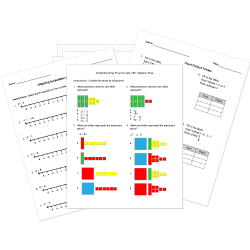Looking for Algebra worksheets?
Check out our pre-made Algebra worksheets!
 Tweet##### Browse Questions
• Arts (658)
• English Language Arts (3081)
• English as a Second Language ESL (1429)
• Health and Medicine (721)
• Life Skills (476)
• Math (2471)

• ### Systems of Equations

• #### Trigonometry

• Physical Education (420)
• Science (7122)
• Social Studies (2157)
• Study Skills and Strategies (13)
• Technology (485)
• Vocational Education (1000)

You can create printable tests and worksheets from these Grade 10 Direct and Inverse Variation questions! Select one or more questions using the checkboxes above each question. Then click the add selected questions to a test button before moving to another page.

Grade 10 Direct and Inverse Variation
Grade 10 Direct and Inverse Variation
When the ratio of two variables is constant, their relationship can be described as
1. inversely proportional.
2. interdependent.
3. directly proportional.
4. parallel.
Grade 10 Direct and Inverse Variation CCSS: HSA-CED.A.2
Grade 10 Direct and Inverse Variation
Grade 10 Direct and Inverse Variation CCSS: HSA-CED.A.2
The amount $x$ varies inversely to $y$. Which equation expresses the given statement?
1. $y=kx$
2. $x=ky$
3. $y=k/x$
4. $-x=k/y$
Grade 10 Direct and Inverse Variation CCSS: HSA-CED.A.2
y varies inversely as the cube of x. If y = 5 when x = 2, find y when x = 6.
1. $5/27$
2. $10/54$
3. $20/108$
4. $1 1/9$
Grade 10 Direct and Inverse Variation CCSS: HSA-CED.A.2
Write the equation to describe the variation: L varies jointly with the square of x and the fourth root of y; L = 9 when x = 3 and y = 1.
1. $L=x^2 root4y$
2. $L=x^2 y^4$
3. $L=root2x root4y$
4. $x=L^2 root4y$
Grade 10 Direct and Inverse Variation CCSS: HSA-CED.A.2
You need to have at least 5 reputation to vote a question down. Learn How To Earn Badges.